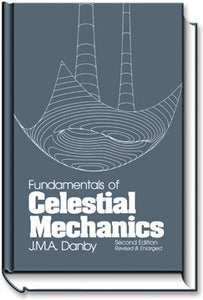# Fundamentals of Celestial Mechanics

• \$30.95
Unit price per

By J.M.A. Danby.

Product Information: hardbound, 466 pages.

Fundamentals of Celestial Mechanics is an introductory text that should be accessible to a reader having a background in calculus and elementary differential equations. The original edition (published in 1962) has been radically revised, and emphasis is placed on computation. The numerical analysis needed for the computations is derived, and sample programs (run on a IBM-PC) are included. There are introductory chapters on the astronomical background and on vectorial mechanics. Sections dealing with the problem of two bodies include the use of universal variables, several methods (including that of Laguerre) for solving Kepler's equation, and three methods for solving the two point boundary value problem. The chapter on the determination of orbits includes two versions of Gauss' method, the application of least squares and an introduction to recursive methods. The chapter on numerical methods has been expanded, and includes three methods for the numerical integration of differential equations, one of which has full stepsize control. There are also chapters on perturbations, the three- and n-body problems, the motion of the Moon and the rotations of the Earth and Moon. The appendix includes numerical tables and derivations of properties of conic sections that are used in the text. The text includes several hundred problems, and suggested computer projects. The computer listings found in the book are available separately, along with a selection of other programs, on CDROM.

The text is accessible to undergraduates or graduate students in mathematics, physics, engineering or astronomy. As minimum requirements, a user is assumed to have taken introductory courses in calculus, differential equations and mechanics. Other topics, such as numerical analysis, are developed in the text as needed. There is plenty of material for two semesters; so a one semester course can be designed in many ways (even with the exclusion of all computing).

WBFCM